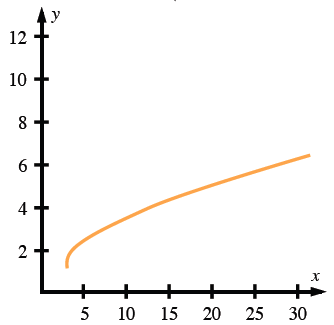### Home > CCA2 > Chapter Ch7 > Lesson 7.1.5 > Problem7-85

7-85.

Find the equation for the inverse of the function $f ( x ) = 2 \sqrt { \frac { ( x - 3 ) } { 4 } } + 1$. Sketch the graph of both the original and the inverse.

To find the inverse, change every $x$ to $f(x)$ and every $f(x)$ to $x$. Solve for $f(x)$.

If you found a few points for the graph of $f(x)$, how can that help you graph the inverse equation?